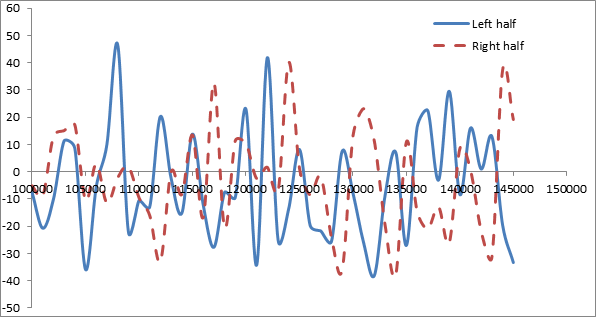# Huge oscillation in the heat flux calculated from compute heat flux

Hi,

I am trying to find out the heat flux through an amorphous polyaniline system using the compute heat flux command. I use real units. I set three regions in my periodic box. One in the middle and 2 in the sides. I have two different cases:

Case 1

I equilibrate everything at 300 K for and then apply Langevin thermostat to set the middle region to 330 and the 2 end regions to 270 for 0.1 ns and use compute heat flux for set of atoms in the right and left half of the system. As a temperature difference of 60 K is established between middle and either ends, I should expect a finite heat flux. However I get highly oscillatory heat flux (I know that the output of compute heat flux gives energy*velocity, however volume remains constant for my case)The average is 0.91 and SD is 18. The attached figure shows the variation of heat flux at left and right half of the material. X axis shows the time steps. Each timestep is 1 femtosecond.Case 2

I do same as case 1 and then unfix the 3 thermostats. So that the system can relax an come back at some equilibrium temperature. Unfortunately, I get the same response . I expected a decaying heat flux!

Why should it fluctuate this much? Can this be controlled through the parameters associated with langevin thermostat or may be some other thermostat should be used? My primary objective is to calculate heat flux through the system in a particular direction by imposing a temperature gradient.

I am also attaching the lammps script for reference. Please tell me if am missing something .

Thanks Souvik.

therm_rnemd.poly (2.04 KB)

You didnt say how big your system was. Do the
oscillations reduce with a bigger system. You
can try running the system discussed in the
example given on the compute heat/flux doc
page and compare. It gives good behavior
for using the heat flux to calculate a thermal
conductivity. You do have to run for a while
to get good statistics for that LJ model.

Steve